## Friday, 16 October 2015

Friends ! Here Find Previous SBI PO data interpretation Test- 1 and Also Take Free Online Quiz practice for SBI PO Preliminary Examinations

Direction (Q. 1 - 5): Study the following graph carefully and answer the questions given below it.
PRODUCTION OF FOODGRAIN OVER THE YEARS (1000 TONNES)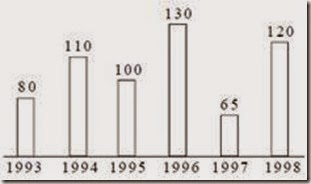1. In the case of how many years was the production below the average production of the given years?
a) one b) two c) three d) four

2. What was the percentage drop in production from 1996 to 1997?
a) 100 b) 50 c) 65 d) 40

3. In which year was the production 50%of the total production in 1993 and 1998 together?
a) 1994 b) 1995 c) 1996 d) 1997

4. If the production in 1999 will be above the average production of the given years, which of the following could be the minimum production for 1999?
a) 105000 b) 120000 c) 100900 d) 130000

5. What was the approximate percentage increase in production from 1993 to 1994
a) 60 b) 40 c) 110 d) 30

Direction (Q. 6 - 10): Study the following graph carefully and answer the questions given below it:
INCOME AND EXPENDITURE OF A COMPANY OVER THE YEARS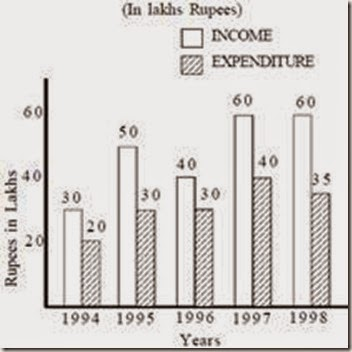6. What was the difference in profit between 1995 and 1996?
a) Rs. 10 lakhs b) Rs. 5 lakhs c) Rs. 15 lakhs d) No profit

7. In the case of how many years was the income more than the average income of the given years?
a) one b) two c) three d) four

8. What was the percentage increase in expenditure from 1996 to 1997?
a) 10 b) 33c) 66d) 20

9. The income in 1996 was equal to the expenditure of which of the following years?
a) 1994 b) 1995 c) 1997 d) 1998

10. In which of the following years was the profit the maximum?
a) 1994 b) 1995 c) 1996 d) 1998

Explanations
1. C; Average production
=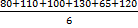=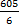= 100.833 thousand tonnes
= 100900 tonnes
ie. production in 1993, 1995 and 1997 was below the average.

2. B; Production in 1996 = 130 thousand tonnes.
Production in 1997 = 65 thousand tonnes
∴ decrease = (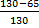) 100
=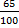x 100 = 50%

3. B; Total production in 1993 and 1998 = 80+120 = 200
50% of 200 =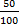x 200 = 100
ie, the production in 1995 was 50% of the total production in 1993 and 1998.

4. C; Average production = 100900 tonnes.
Therefore the minimum production in 1999 will be 100900 tonnes

5. B; Production in 1993 = 80 thousand tonnes.
Production in 1994 = 110 thousand tonnes Increase
= (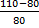) 100 =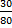x 100 = 40%

6. A; Profit in 1995 = 50-30=Rs. 20 lakhs
Profit in 1996 = 40-30 = Rs. 10 lakhs
Difference in profit=20-10=Rs. 10 lakhs

7. C; Average income
=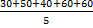=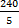= 48
Therefore in 1995, 1997 and 1998, the income is more than the average income

8. B; Expenditure in 1996 = Rs. 30 lakh
Expenditure in 1997 = Rs. 40 lakh
Increase
= (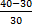) 100 =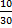x 100 = 33%

9. C; The income in 1996 was equal to the expenditure in 1997 ie Rs. 40 lakhs.

10. D; The profit is maximum in the year 1998.

Install Android App for IBPS Exam Guru